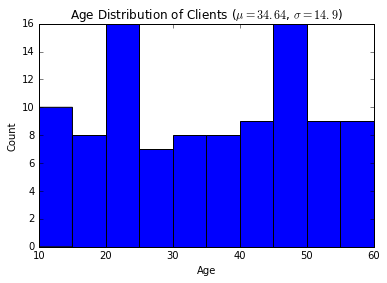# Basic Plots¶

The main tool for plotting in Python is the Matplotlib package. If you are familiar with MATLAB plotting, then Matplotlib will be easy to use for basic plotting. Its documentation can be a bit tricky to navigate if you want to do more than basic plotting. There are lots of examples on matplotlib website. To understand most, you have to know some basic Matplotlib terminology and organization. To help you learn how to use Matplotlib effectively for more than basic plotting, here we will collect some simple examples and add tasks beyond basic plotting incrementally. Also, given below is a guide to the Matplotlib documentation including the order in which you should browse the documentation.

## Pylab, Pyplot, and object-oriented interfaces to Matplotlib¶

Plotting in Matplotlib can be accessed either via the Pylab interface, the Pyplot interface or via an object-oriented class hierarchy. For non-programmers, or for MATLAB experts, the first two methods may be more comfortable. The Pyplot interface provides MATLAB-like commands for plotting. The Pylab interfaces provides these and in addition imports the NumPy package for convenience. Since we are doing all our work in IPython which imports the top level NumPy functionality anyway, we will use the Pyplot interface when we are not using the object-oriented interface.

Ignore the following line -- it is somethng needed for Jupyter notebooks.

In :
%matplotlib inline


## Draw a simple plot¶

Let us start by using the Pyplot interface to make some plots. Plot the $\sin$ function in $[-2\pi, 2\pi]$. Note the show command at the end. You need that to actually display anything produced by matplotlib.

In :
from math import pi
import matplotlib.pyplot as plt
from numpy import sin, linspace

x = linspace(-2*pi, 2*pi, 100)
plt.plot(x, sin(x))
plt.show() # your need the show command to actually see the plot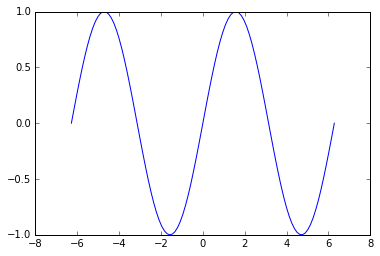Change the vertical limits so that there is some space between the plot and the axis box.

In :
from math import pi
import matplotlib.pyplot as plt
from numpy import sin, linspace

x = linspace(-2*pi, 2*pi, 100)
plt.plot(x, sin(x))
plt.ylim([-1.5, 1.5])
plt.show()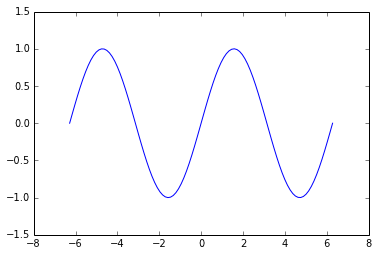It would be nice to be able to see the $x$ and $y$ axes. Let's turn those on.

In :
from math import pi
import matplotlib.pyplot as plt
from numpy import sin, linspace

x = linspace(-2*pi, 2*pi, 100)
plt.plot(x, sin(x))
plt.ylim([-1.5, 1.5])
plt.axhline(); plt.axvline()
plt.show()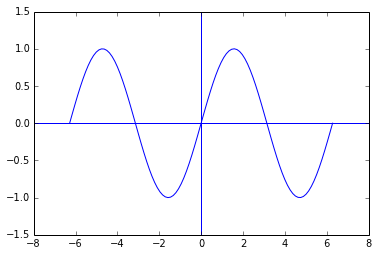It is distracting to have the axes and plot be in the same color. We can change any of these. Let's change the color of the axes to black.

In :
from math import pi
import matplotlib.pyplot as plt
from numpy import sin, linspace

x = linspace(-2*pi, 2*pi, 100)
plt.plot(x, sin(x))
plt.ylim([-1.5, 1.5])
plt.axhline(color='k'); plt.axvline(color='k')
plt.show()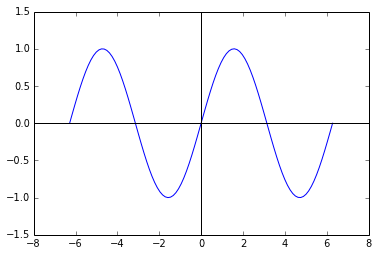The axes can be placed anywhere. Explore the possibile variations by typing ?plt.axhline at the ipython prompt. Instead let's do some other simple things. Say you want to get rid of the box surrounding the figure. Use the box() command to toggle state or box('on'), box('off'), or use a boolean argument.

In :
from math import pi
import matplotlib.pyplot as plt
from numpy import sin, linspace

x = linspace(-2*pi, 2*pi, 100)
plt.plot(x, sin(x))
plt.ylim([-1.5, 1.5])
plt.axhline(color='k'); plt.axvline(color='k')
plt.box()
plt.show()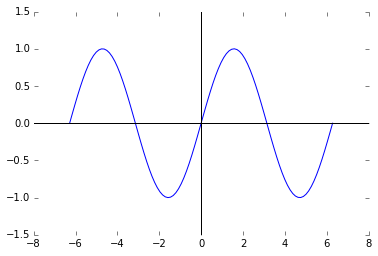This looks ugly. Let's get rid of the axes completely.

In :
from math import pi
import matplotlib.pyplot as plt
from numpy import sin, linspace

x = linspace(-2*pi, 2*pi, 100)
plt.plot(x, sin(x))
plt.ylim([-1.5, 1.5])
plt.axhline(color='k'); plt.axvline(color='k')
plt.axis('off')
plt.show()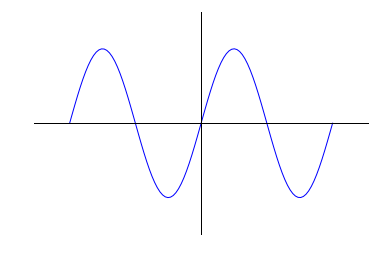Let's go back to the box axes and remove the black axes intersecting at the origin.

In :
from math import pi
import matplotlib.pyplot as plt
from numpy import sin, linspace

x = linspace(-2*pi, 2*pi, 100)
plt.plot(x, sin(x))
plt.ylim([-1.5, 1.5])
plt.show()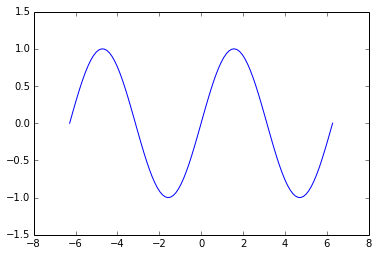The above plots are smooth looking plot which don't show the sample points. It is possible to make such plots using collection of points (markers). It is also possible to combine a continuous plot and a plot of the markers. The following example shows how to do this. It also shows how to create separate figures.

In :
from math import pi
import matplotlib.pyplot as plt
from numpy import sin, linspace

x = linspace(-2*pi, 2*pi, 30)
plt.ylim(-1.5, 1.5)
plt.plot(x, sin(x), 'ro')
plt.figure()
plt.ylim(-1.5, 1.5)
plt.plot(x, sin(x), 'ro')
plt.plot(x, sin(x), 'k-')

Out:
[<matplotlib.lines.Line2D at 0x10cb7e790>]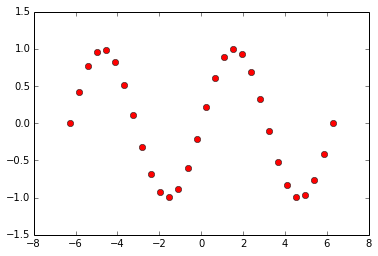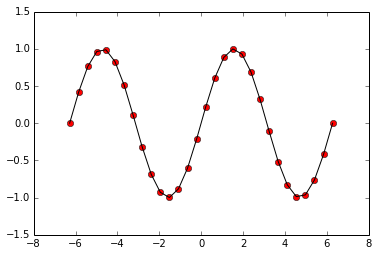In :
from math import pi
from matplotlib.pylab import plot, show, title, xlabel, \
ylabel
from numpy import sin, linspace

x = linspace(-2*pi, 2*pi, 30)
plot(x, sin(x), 'k-')
plot(x, sin(x), 'ro')
title('$\sin(\\theta)$')
xlabel('$\\theta$')
ylabel('$\sin(\\theta)$')

Out:
<matplotlib.text.Text at 0x10c339a90>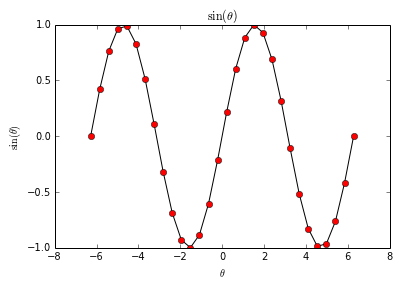In :
from math import pi
from matplotlib.pylab import plot, show, title, xlabel, \
ylabel
from numpy import sin, linspace

x = linspace(-2*pi, 2*pi, 30)
plot(x, sin(x), 'k-')
plot(x, sin(x), 'ro')
title('$\sin(\\theta)$', fontsize=20)
xlabel('$\\theta$', fontsize=20)
ylabel('$\sin(\\theta)$', fontsize=20)
grid('on')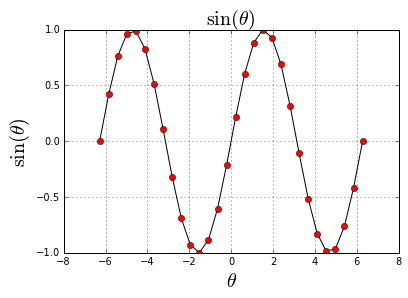## Basic data analysis¶

In an earlier module (files-string we looked at reading, processing and structuring data from files. We will not do some very rudimentary analysis and plotting of that data.

For example, we may want a histogram of the clients' ages or a description of the mean and standard deviation of the clients' ages.

Further, we don't just want a list of numbers but some kind of a visual representation of the dataset.

Let's start out with visualizing our data, since pictures are fun to make.

We will process the client data again, but this time only extracting their ages.

In :
def parse(line):
first, last, age, phone = line.split()
return int(age)

ages = [parse(line) for line in open('clients.txt')]


Now, let's see how to create a basic histogram - nothing fancy.

In :
%matplotlib inline
import matplotlib.pyplot as plt

plt.figure()
plt.hist(ages)
plt.show()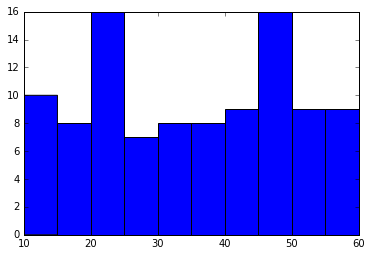Now, since we created this plot, we understand what we're looking at, but to someone who comes across this randomly, it's not very useful. Let's see if we can add some context to it.

In :
plt.figure()
plt.title('Age Distribution of Clients')
plt.xlabel('Age')
plt.ylabel('Count')
plt.hist(ages)
plt.show()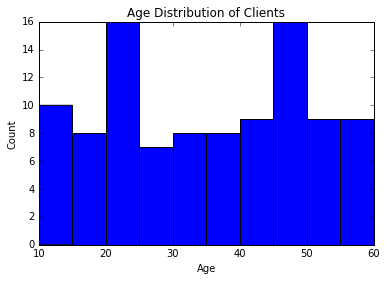If we're happy with the plot, we can save it for later use using the savefig command instead of show.

In :
plt.figure()
plt.title('Age Distribution of Clients')
plt.xlabel('Age')
plt.ylabel('Count')
plt.hist(ages)
plt.savefig('age-histogram.png')Now we've gotten some visual feedback of what our distribution looks like. It looks like it has a mean around 35 and a large variance. To quantify this, let's use Scipy's describe function.

In :
from scipy.stats import describe
info = describe(ages)
info

Out:
DescribeResult(nobs=100, minmax=(10, 60), mean=34.640000000000001, variance=222.11151515151516, skewness=-0.010473771030976402, kurtosis=-1.2898171636853601)

Let's try to add this newfound information to our plot!

In :
mu = round(info.mean, 2)
sigma = round(info.variance**.5, 2)

plt.figure()
plt.title('Age Distribution of Clients ($\\mu={}$, $\\sigma={}$)'.format(mu, sigma))
plt.xlabel('Age')
plt.ylabel('Count')
plt.hist(ages)
plt.savefig('age-histogram.png')

# The \\ is needed in the title string as \ performs special "escape" commands. For example,
# we've seen that \n produces a newline. \\ just leaves us with a \